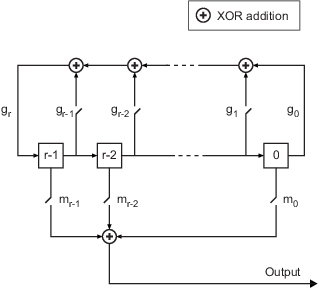Convert shift to mask vector for shift register configuration

## Syntax

```mask = shift2mask(prpoly,shift) ```

## Description

`mask = shift2mask(prpoly,shift)` returns the mask that is equivalent to the shift (or offset) specified by `shift`, for a linear feedback shift register whose connections are specified by the primitive polynomial `prpoly`. The `prpoly` input can have one of these formats:

• A binary vector that lists the coefficients of the primitive polynomial in order of descending powers

• An integer scalar whose binary representation gives the coefficients of the primitive polynomial, where the least significant bit is the constant term

The `shift` input is an integer scalar.

Note

To save time, `shift2mask` does not check that `prpoly` is primitive. If it is not primitive, the output is not meaningful. To find primitive polynomials, use `primpoly` or see .

The equivalent mask for the shift s is the remainder after dividing the polynomial xs by the primitive polynomial. The vector `mask` represents the remainder polynomial by listing the coefficients in order of descending powers.

### Shifts, Masks, and Pseudonoise Sequence Generators

Linear feedback shift registers are part of an implementation of a pseudonoise sequence generator. Below is a schematic diagram of a pseudonoise sequence generator. All adders perform addition modulo 2.The primitive polynomial determines the state of each switch labeled gk, and the mask determines the state of each switch labeled mk. The lower half of the diagram shows the implementation of the shift, which delays the starting point of the output sequence. If the shift is zero, the m0 switch is closed while all other mk switches are open. The table below indicates how the shift affects the shift register's output.

T = 0T = 1T = 2...T = sT = s+1
Shift = 0x0 x1 x2 ...xs xs+1
Shift = s > 0xs xs+1 xs+2 ... x2s x2s+1

If you have Communications Toolbox™ software and want to generate a pseudonoise sequence in a Simulink® model, see the PN Sequence Generator block reference page.

## Examples

collapse all

Convert a shift in a linear feedback shift register into an equivalent mask.

Convert a shift of 5 into the equivalent mask ${x}^{3}+x+1$ for the linear feedback shift register whose connections are specified by the primitive polynomial ${x}^{4}+{x}^{3}+1$. The length of the mask is equal to the degree of the primitive polynomial, 4.

`mk = shift2mask([1 1 0 0 1],5)`
```mk = 1×4 1 0 1 1 ```

Convert a shift of 7 to a mask of ${x}^{4}+{x}^{2}$ for the primitive polynomial ${x}^{5}+{x}^{2}+1$.

`mk2 = shift2mask('x5+x2+1',7)`
```mk2 = 1×5 1 0 1 0 0 ```

## References

 Lee, J. S., and L. E. Miller, CDMA Systems Engineering Handbook, Boston, Artech House, 1998.

 Simon, Marvin K., Jim K. Omura, et al., Spread Spectrum Communications Handbook, New York, McGraw-Hill, 1994.

## Version History

Introduced before R2006a